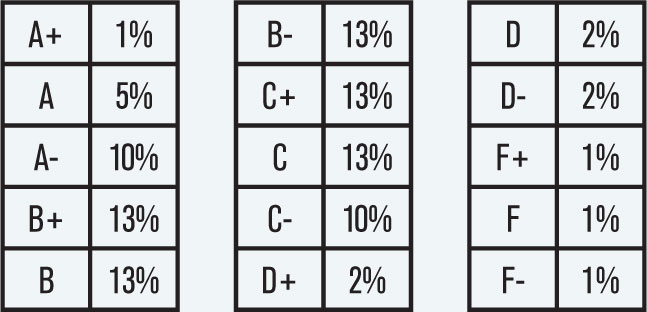# Bending a Laser Beam

Dr. Snell is an expert in applied optical physics at the patent review office. He receives a patent application (figure below) for an optical element in the shape of a 90-degree arc of a translucent annulus.

The application claims that this device can gradually bend a laser beam entering Side A by a total of 90 degrees upon exit from Side B. The mechanism of the bending works on the premise that the index of refraction of the material inside the device, N(θ), is a continuous and smooth function of angle N(θ). What do you think Dr. Snell’s opinion will be as to whether this device can work? If he thinks it can work, what is the function, or possible different functions, for N(θ)?Professor Mannboltz was hired to calibrate the ranges of numeric scores assigned to letter grades on a new standardized exam, which will be scored from 0 to 2,400 points. Without any information about actual scores except that the average score on an exam like this is 30% of the points, Mannboltz had to target the following percentages of students for each grade:A good way to solve a problem like this, in the tradition of Professor Mannboltz’s specialty of statistical mechanics, is through maximum entropy distributions, as described in Lewis H. Roberts’s Proceedings article, “A Discipline for the Avoidance of Unnecessary Assumptions.”*

There are many probability distributions on the possible scores from 0 to 2,400, that average 720, or 30% of the possible points. In the absence of any other information, it makes sense to pick the distribution with the least additional information, the maximum entropy distribution with average 720. The general form for the probability density of a maximum entropy distribution given its mean is P(x)=ec0+c1x . The constant c0 is uniquely determined by the normalization requirement for any choice of the constant c1. Assuming 2,401 discrete possible scores, the integers from 0 to 2,400, we can search (through calculations in Excel, R, etc.) for the value of c1 that gives a mean of 720. The values are approximately c0=-6.730133071 and c1=-0.001112339. Summing up the probabilities for the discrete score intervals suggests the following grade ranges, given the previous targets for percentages of students in each range:

 F- 0 – 7 F 8 – 15 F+ 16 – 24 D- 25 – 41 D 42 – 59 D+ 60 – 77 C- 78 – 173 C 174 – 316 C+ 317 – 486 B- 487 – 696 B 697 – 971 B+ 972 – 1,367 A- 1,368 – 1,868 A 1,869 – 2,286 A+ 2,287 – 2,400

Note: We could have reached a similar solution by modeling the scores as a continuous distribution. With a continuous approach, however, it is best to allow the point range for the distribution to be the interval from -0.5 to 2,400.5 in order to equitably account for the actual minimum score of 0 and actual maximum score of 2,400.

Solutions were also submitted by Bob Conger, Rob Kahn and Stephen Mildenhall.

* Roberts, Lewis H., PCAS, LIV, 1967.
Roberts, p.214: “[G]iven only the mean of a non-negative variable, we know the exponential distribution is the minimally prejudiced estimate of the distribution.”